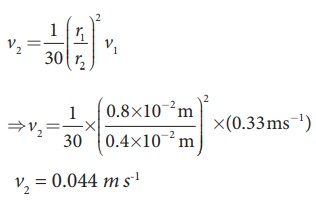Home | | Physics 11th std | Solved Example Problems for BernoulliŌĆÖs Theorem, Equation of continuity

# Solved Example Problems for BernoulliŌĆÖs Theorem, Equation of continuity

Physics : Properties of Matter - Solved Example Problems for BernoulliŌĆÖs Theorem, Equation of continuity

## EXAMPLE 7.14

In a normal adult, the average speed of the blood through the aorta (radius r = 0.8 cm) is 0.33 ms-1. From the aorta, the blood goes into major arteries, which are 30 in number, each of radius 0.4 cm. Calculate the speed of the blood through the arteries.

## Solution:

a1v1 = 30 a2 v2 ŌćÆ ŽĆ r12v1  = 30 ŽĆ r22v2Study Material, Lecturing Notes, Assignment, Reference, Wiki description explanation, brief detail
11th Physics : UNIT 7 : Properties of Matter : Solved Example Problems for BernoulliŌĆÖs Theorem, Equation of continuity |